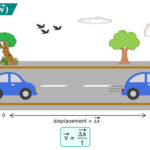Home / Physics / Electric Potential Energy

# Electric Potential Energy

Consider an electric field generated by a positive point charge. The direction of the electric field is such that it is radially outwards. When a positive test charge is brought closer to the point charge, it will experience repulsion due to electrostatic or Coulomb force. Energy is needed to overcome the repulsive force and move the test charge closer to the point charge, which is a source charge. This energy is known as electric potential energy.

## Electrical Potential Energy Formula

The electric potential energy is a scalar quantity. It is not a vector, although the electric field responsible for it is a vector. The magnitude depends upon two factors:

1. The magnitude of the charges
2. Distance between the two charges

Suppose q1and q2are the magnitudes of the two charges and r is the separation distance between them. Then, the electric potential energy U is given by

U ∝ q1q2/r

Or, U = kq1q2/r

Where k is a proportionality constant known as Coulomb constant, given by k = 1/(4πεo), whose value is 9 x 109 N m2/C2.

SI Unit: Joule (J)

Dimension: [ML2T-2]

Symbol: U

The electric potential energy per unit charge is known as electric potential.

It can be obtained by dividing the electric potential energy by the magnitude of the test charge.

V = U/q1

Or, V = kq1/r

Replacing k by 1/(4πεo) and q1 by Q, we get the formal expression of the electric potential.

V = Q/(4πεor)

Unit: Volt (V) or Joule/Coulomb (J/C)

Dimension: [ML2T-3A-1]

Symbol: V

The above equation gives the electric potential at a distance r from the source charge Q. If this charge is negative, the electric potential is negative and given by

V = -Q/(4πεor)

Work and Electric Potential

Suppose a unit charge is moved from point A to B such that B is closer to the source charge than A. Let rA and rB represent the distances of A and B from Q. Then, rA> rB. The potential difference between two points, A and B, can be written as

ΔV = VB – VA = (Q/4πεo)(1/rB – 1/rA)

Work is done against the electric field to move the unit charge from A to B. The work done is the negative change in electric potential.

W = – ΔV

The work done is negative because the displacement is opposite to the electric field. In other words, the charge is displaced in a direction opposite to the electric field.

The electric potential at a point is said to be one volt if one joule of work moves one Coulomb of the electric charge against the electric field.

Zero Potential

The zero potential is a reference point from which electric potential values are measured. For a point charge, it is clear from the above equation that the electric potential is zero at infinity. This point is taken as a reference point. Hence, work done is the change in electric potential when a unit charge is brought from infinity to a point under consideration. Alternatively, the electric potential at a point is the work done in moving a unit charge from infinity to that point.

The fundamental difference between electric potential energy and electric potential is that the former is the energy required to move an electric charge against an electric field. On the other hand, the latter is the work done in moving a unit charge from infinity to the point under consideration.

## Example Problems and Solutions

Problem 1: Two charges of magnitude 2 nC and 3 nC are placed at 2 cm from each other. Calculate the electric potential energy between these two charges.

Solution:

Given

q1 = 2 nC

q2 = 3 nC

r = 2 cm = 0.02 m

The electric potential energy is given by

U = kq1q2/r

Or, U = (9 x 109 Nm2C-2 x 2 x 10-9 C x 2 x 10-9 C)/0.02 m

Or, U = 2.7 x 10-6J

Problem 2: A +2 μC test charge is initially at rest a distance of 15 cm from a +7 μC source charge fixed at the origin. The Coulomb force pushes the test charge away from the source charge, reaching 20 cm. What is the work done by the electric field?

Solution:

Given

q1 = +2 μC

q2= +7 μC

rA = 15 cm = 0.15 m

rB = 20 cm = 0.20 m

The work done is given by

W = -ΔV

Or, W = -(kq1q2)(1/rB – 1/rA)

Or, W = -(9 x 109 Nm2C-2 x 7 x 10-6 C x 2 x 10-6 C)(1/0.20 m- 1/0.15 m)

Or, W = 0.21 J

Problem 2: Two charges of magnitude 2 nC and 3 nC are placed at 2 cm from each other. Calculate the electric potential energy between these two charges.

Solution:

Given

q1 = 2 nC

q2 = 3 nC

r = 2 cm = 0.02 m

The electric potential energy is given by

U = kq1q2/r

Or, U = (9 x 109 Nm2C-2 x 2 x 10-9 C x 2 x 10-9 C)/0.02 m

Or, U = 2.7 x 10-6J

## FAQs

Q.1. What is the difference between electric potential and electric field?

Ans. The difference between the electric potential and electric field is that the former is the work done in moving a charge from infinity to a point under consideration. On the other hand, the electric field is the electric force per unit charge.

Article was last reviewed on Monday, July 4, 2022

### Related articlesLaminar FlowRadius of GyrationNewton’s Law of CoolingVelocity